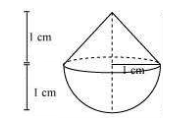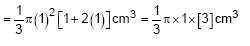Newbie

# A solid is in the shape of a cone standing on a hemisphere with both their radii being equal to 1 cm and the height of the cone is equal to its radius. Find the volume of the solid in terms of π. Q.1

• 0

Find the solution of the chapter surface areas and volume of ncert class 10 , give me the simplest method of the exercise 13.2 question number 1 . Find the best and easy solution of the question also give easiest solution of this question.A solid is in the shape of a cone standing on a hemisphere with both their radii being equal to 1 cm and the height of the cone is equal to its radius. Find the volume of the solid in terms of π.

Share

1. Here r = 1 cm and h = 1 cm.

The diagram is as follows.Now, Volume of solid = Volume of conical part + Volume of hemispherical part

We know the volume of cone = ⅓ πr2h

And,

The volume of hemisphere = ⅔πr3

So, volume of solid will be= π cm3

• 0